Chapter 10
Machine Learning in the Cloud
“Learning is any change in a system that produces a more or less
permanent change in its capacity for adapting to its environment.
—Herbert Simon, The Sciences of the Artiﬁcial
Machine learning has become central to applications of cloud computing. While
machine learning is considered part of the ﬁeld of artiﬁcial intelligence, it has roots
in statistics and mathematical optimization theory and practice. In recent years it
has grown in importance as a number of critical appl icati on breakthroughs have
taken place. These include human-quality speech recognition [
144
] and real-time
automatic language translation [
95
], computer vision accurate and fast enough to
propel self-driving cars [
74
], and applications of rei nforcement learning tha t allow
machines to master some of the mos t com plex human games, such as Go .
What has enabled these breakthroughs has been a convergence of th e availabil-
ity of big data plus algorithmic advances and faster computers that have made it
possible to train even deep neural networks. The same technology is now being
applied to scientiﬁc prob lem s as diverse as predicting protein structure [
180
], pre-
dicting the pharmacological properties of drugs [
60
], and identifying new materials
with desired properties .
In this chapter we introduce some of the major machine learning tools that are
available in public clouds, as well as toolkits that you can install on a private cloud.
We begin with our old friend Spark and its machine learning (ML) package, and
then move to Azure ML. We progress from the core “classical” ML tools, including
logistic regression, clustering, and random forests, to take a brief look at deep
learning and deep learning toolkits. Given our emphasis on P ython, the reader may10.1. Spark Machine Learning Library (MLlib)
expect u s to cover the excellent Python library scikit-learn. However, scikit-learn
is well covered elsewhere [
253
], and we introduced several of its ML methods in our
microservice-based science document classiﬁer example in chapter 7. We describe
the same example, but using dierent technology, later in this chapter.
10.1 Spark Machine Learning Library (MLlib)
Spark MLlib [
198
], sometimes referred to as Spark ML, provides a set of high-level
APIs for creating ML pipelines. It implements four basic concepts.
DataFrames
are containers created from Spark RDDs to hold vectors and
other structured types in a manner that permits ecient execution [
45
]. Spark
DataFrames are similar to Pandas DataFrames and share some operations.
They are distributed objects that are part of the execution graph. You can
convert them to Pandas DataFrames to acces s them in Python.
Transformers
are operators that convert one DataFrame to another. Since
they are nodes on the execution graph, they are not evaluated until the entire
graph is executed.
Estimators
encapsulate ML and other algorithms. As we describe in the
following, you can use the
fit(...)
method to pass a D ataFrame and
parameters to a learning algorithm to create a model. The model is now
represented as a Trans former.
A
Pipeline
(usually linear, bu t can be a directed acyclic graph) l inks Trans-
formers and Estim ators to specify an ML workﬂow. Pipelines inherit the
fit(...)
method from the contained estimator. Once the estimator is
trained, the pipeli ne is a model and has a
transform(...)
method that can
be used to push new cases through the pipeline to make predictions.
Many transformers exist, for example to turn text documents into vectors of
real numbers, convert columns of a DataFrame from one form to another, or split
DataFrames into subsets. There are also various kinds of estimators, ranging from
those that transform vectors by projecting them onto principal component vectors,
to
n
-gram generators that take text documents and return strings of
n
consecutive
words. Classiﬁcation models inclu de logistic regression, decision tree classiﬁers,
random forests, and naive Bayes. The family of clustering methods includes
k
-
means and latent Dirichlet allocation (LDA). The MLlib online documentation
provides much useful material on these and related topics  .
192Chapter 10. Machine Learning in the Cloud
10.1.1 Logistic Regression
The example that follows employs a metho d called
logistic regression
[
103
],
which we introduce here. Suppose we have a set of feature vectors
x
i
2 R
n
for
i
in [0
,m
]. Associated with each feature vector is a binary outcome
y
i
.Weare
interested in the cond itio nal probability
P
(
y
=1
|x
), which we approximate by a
function
p
(
x
). Becaus e
p
(
x
) is between 0 and 1, it is not expressible as a linear
function of
x
, and thus we cannot use regular linear regression. Instead, we look
at the “odds” expression p(x)/(1 p(x)) and guess that its log is linear. That is:
ln
p(x)
1 p(x)
= b
0
+ b · x,
where the oset
b
0
and the vector
b
=[
b
1
,b
2
,...b
n
] deﬁne a hyperplane for linear
regression. Solving this expression for p(x) we obtain:
p(x)=
1
1+e
(b
0
+b·x)
We then predict
y
=1if
p
(
x
)
>
0 and zero otherwise. Unfortunately, ﬁnding
the best
b
0
and
b
is not as easy as in the case of linear regression. However, s imp le
Newton-like iterations converge to good solutions if we have a sample of the feature
vectors and known outcomes.
(We note that the logistic function (t) is deﬁned a s fol lows:
(t)=
e
t
e
t
+1
=
1
1+e
t
It is used frequently in machine learning to map a real number into a probability
range [0, 1]; we use it for this purpose la ter in this chapter.)
10.1.2 Chicago Restaurant Example
To illustrate the use of Spark MLlib, we apply it to an example from the Azure
HDInsight tutorial [
195
], na mely predicting whether restaurants pass or fail health
inspections based on the free text of an inspector’s comments. We provide two
versions of this example in notebook 18: the HDInsight version and a version that
runs on any generic Spark deployment. We present the second here.
The data, from the City of Chicago Data Portal
data.cityofchicago.org
,are
a set of restaurant heath inspection reports. Each inspection report contains a
report number, the name of the owner of the establishment, the name of the
193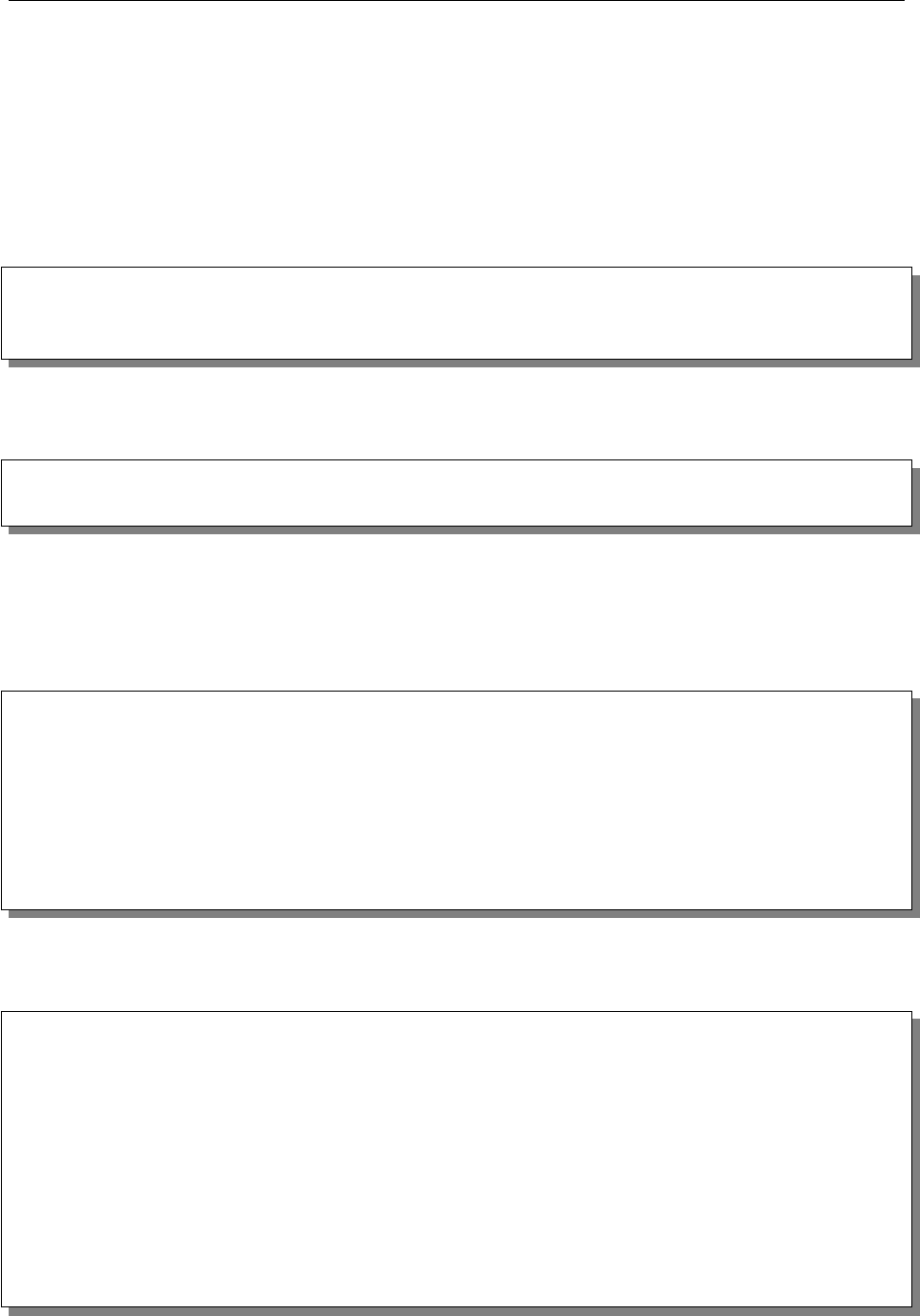10.1. Spark Machine Learning Library (MLlib)
establishment, the address, and an outcome (“Pass,” “Fail,” or some alternative
such as “out of business” or “not available”). It also contain s the (free-text) English
We ﬁrst read the data. If we are using Azure HDInsight, we can load it from
blob storage as follows. We use a simple function
csvParse
that takes each line in
the CSV ﬁle and parses it using Python’s csv.reader() function.
inspections = spark.sparkContext.textFile( \
' wasb :/// HdiSamples /HdiSamples /FoodInspectionData /
Food_Inspections1.csv'). map(csvParse)
The version of the program in notebook 18 uses a slightly reduced dataset. We
have eliminated the address ﬁelds and some other data that we do not use here.
inspections = spark.sparkContext.textFile(
'/path-to-reduced-data/Food_Inspections1.csv' ). map(csvParse)
We want to create a
training set
from a set of inspection reports that contain
outcomes, for use in ﬁtting our logistic regression model. We ﬁrst convert the RDD
containing the data,
inspections
, to create a DataFrame,
df
, with four ﬁelds:
record id, restaurant name, inspection result, and any recorded violations.
schema = StructType ([ StructField ("id", IntegerType (), False),
StructField("name", StringType(), False),
StructField("results", StringType(), False),
StructField("violations", StringType (), True)])
df = spark .creat eData Frame( inspections . map (\
lambda l: (int(l), l, l, l)) , schema)
df . re gister TempTa ble ( ' CountResults')
If we want to look at the ﬁrst few elements, we can apply the
show()
function
to return values to the Python environment.
df . show(5)
+-------+--------------------+---------------+--------------------+
| id |name|results|violations|
+-------+--------------------+---------------+--------------------+
|1978294| KENTUCKY FRIED CH...| Pass |32. FOOD AND NON -...|
|1978279| SOLO FOODS|Out of Business | |
|1978275| SHARKS FISH & CHI...| Pass |34. FLOORS: CONST ...|
|1978268| CARNITAS Y SUPERM...| Pass |33. FOOD AND NON -...|
|1978261| WINGSTOP| Pass| |
+-------+--------------------+---------------+--------------------+
only showing top 5 rows
194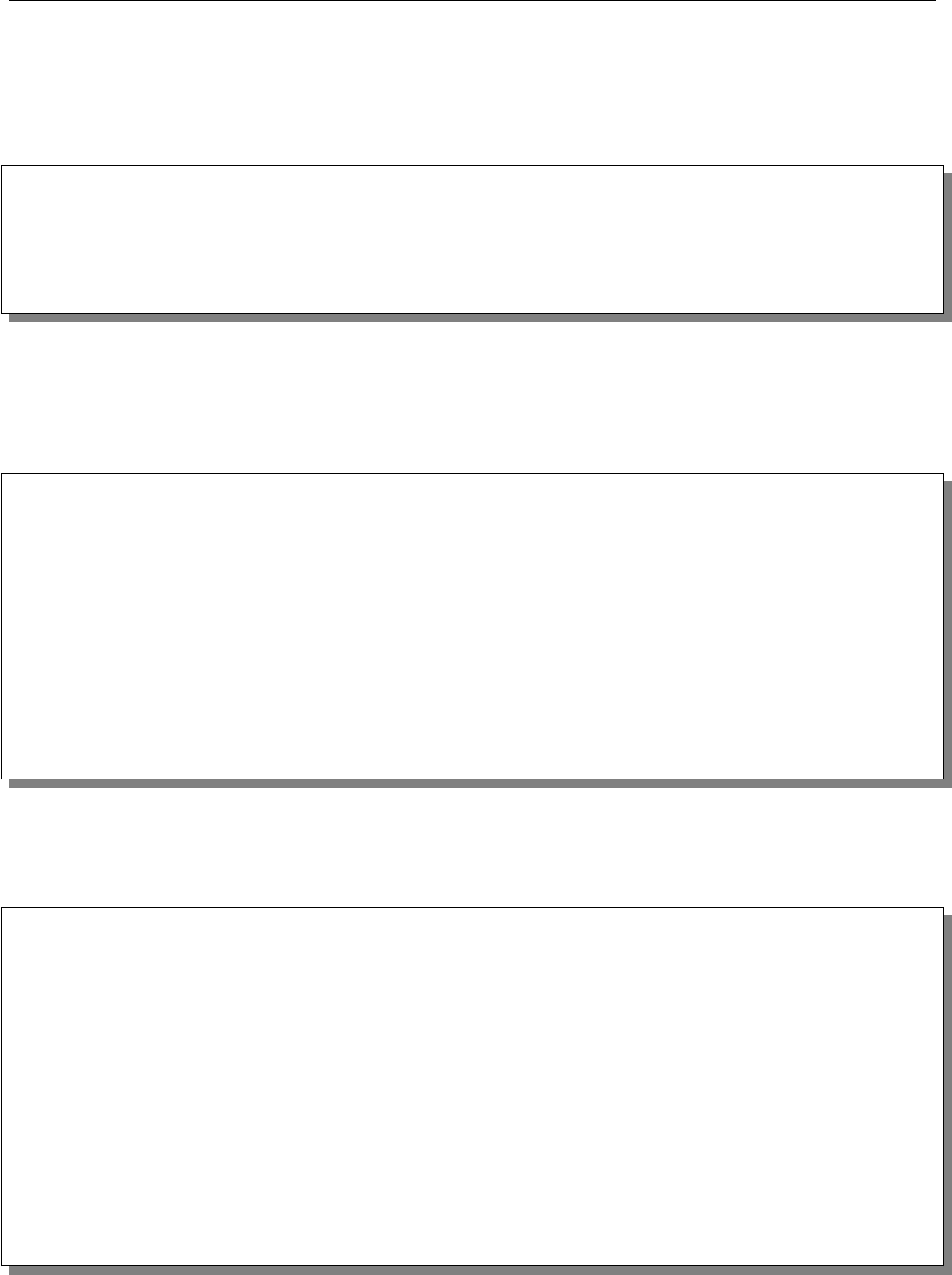Chapter 10. Machine Learning in the Cloud
Fortunately for the people o f Chicago, it seems that the majority of the
inspections result in passing grades. We can use some DataFrame operations to
count the passing and failing grades.
print("Passing = %d"%df[df.results == ' Pass '].count())
print("Failing = %d"%df[df.results == ' Fail '].count())
Passing = 61204
Failing = 20225
To train a logi stic regression model, we need a DataFrame with a bin ary label
and feature vector for each record. We do not want to use records associated with
“out of business” or other special cases, so we map “Pass” and “Pass with conditions”
to 1, “Fail” to 0, and all others to -1, whi ch we ﬁlter out.
def labelForResults(s):
if s==' Fail ':
return 0.0
elif s==' Pass w/ Conditions ' or s==' Pass ':
return 1.0
else:
return -1.0
label = UserDefinedFunction (labelForResults , DoubleType ())
labeledData = df.select(label(df.results ).alias('label'), \
df . violations).where ( ' label >= 0')
We now have a DataFrame with two columns,
label
and
violations
and we
are ready to create and run the Spark MLlib pipeline that we will use to train our
logistic regression model, which we do with the following code.
# 1) Define pipeline components
# a) Tokenize ' violations' and place result in new column ' words '
tokenizer = Tokenizer (inputCol=" violations", outputCol="words")
# b) Hash ' words ' to create new column of ' features '
hashingTF = HashingTF (inputCol="words" , outputCol="features")
# c) Create instance of logistic regression
lr = Log isticR egress ion ( maxIter=10 , regParam=0.01)
# 2) Construct pipeline: tokenize , hash , logistic regression
pipeline = Pipeline(stages =[tokenizer , hashingTF , lr])
# 3) Run pipeline to create model
model = pipeline.fit(labeledData )
We ﬁrst (1) deﬁne our three pipeline components, which (a) tokenize each
violations
entry (a text string) by reducing it to lower case and splitting it into
195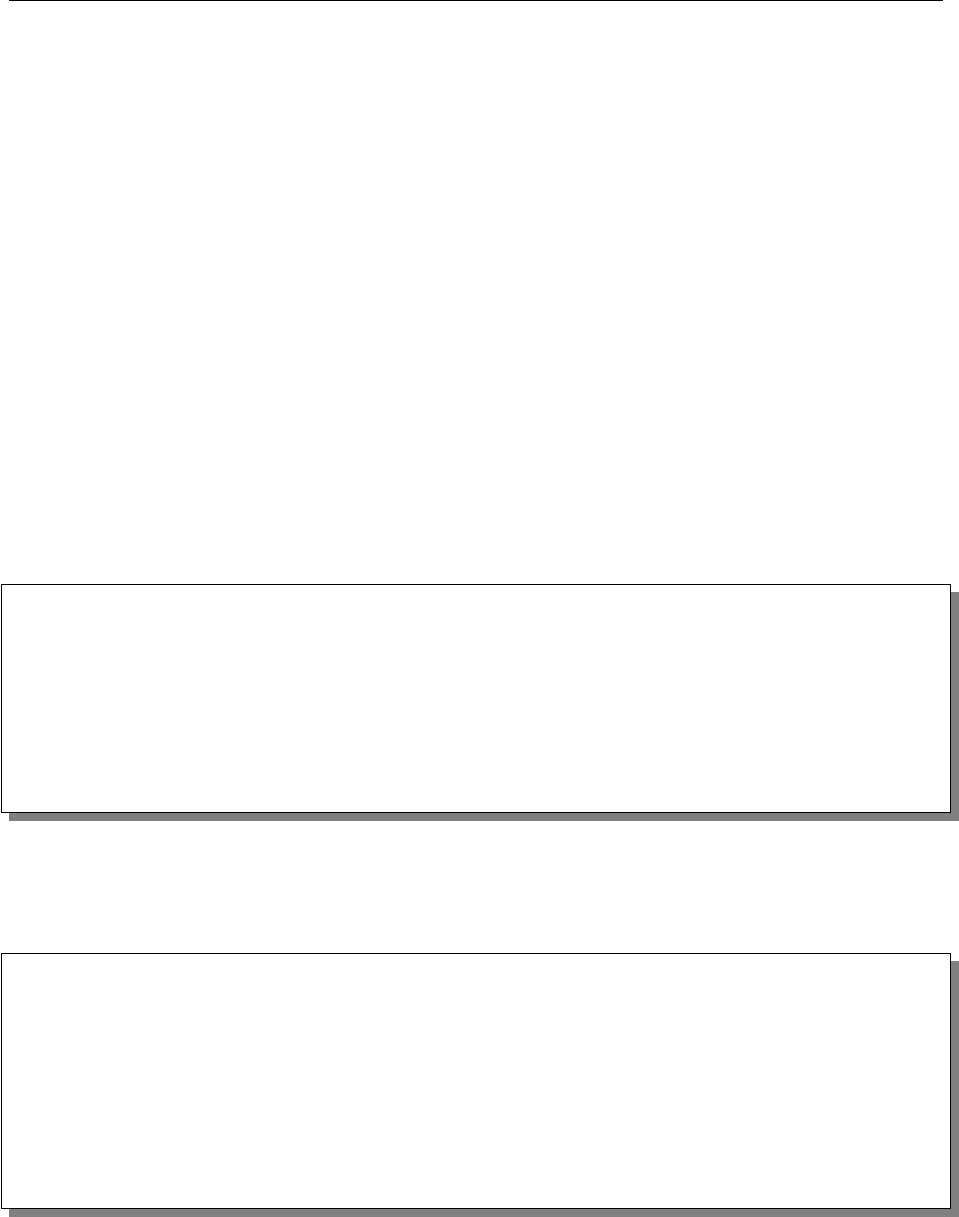10.1. Spark Machine Learning Library (MLlib)
a vector of words; (b) convert each word vector into a vector in
R
n
for some
n
,
by applying a hash function to map each word token into a real number value
(the new vectors have length equal to the size of the vocabulary, and are stored as
sparse vectors); and (c) create an instance of l ogi stic regression. We then (2) put
everything into a pipeline and (3) ﬁt the model with our labeled data.
Recall that Spark implements a graph execution model. Here, the pipeline
created by the Python program is the graph; this graph is passed to the Spark
execution engine by calling the
fit(...)
method on the pipeline. Notice that
the
tokenizer
words
to our working DataFrame, and
hashingTF
features
; thus, the working DataFrame has columns
ID, name, results, label, violations, words, features
when logistic re-
gression is run. The names a re important, as logistic regression looks for columns
label, features
, which it uses for training to build the model. The trainer is
iterative; we give it 10 iterations and an algorithm-dependent value of 0.01.
We can now test the model with a separate tes t collection as follows.
testData = spark.sparkContext.textFile(
'/data_path/Food_Inspections2.csv')\
. map (csvParse) \
. map ( lambda l: (int(l), l, l, l))
testDf = spark. createDataFrame( testData , schema ).
where("results = ' Fail ' OR results = 'Pass' OR \
results = ' Pass w/ Conditions '")
predictionsDf = model.transform(testDf)
The logistic regression model has appended several new columns to the data
frame, including one called
prediction
. To test our prediction success rate, we
compare the prediction column with the results column.
numSuccesses = predictionsDf.where(\
"""( prediction = 0 AND results = ' Fail ') OR \
( prediction = 1 AND ( results = ' Pass ' OR \
results = ' Pass w/ Conditions '))""" ). count()
numInspections = predictionsDf.count()
print("There were %d inspections and there were %d predictions"\
%( numInspections , num Successes ))
print("This is a %2.2f sucess rate"\
%( float (numSuccesses) / float (numInspections) * 100))
We see the following output:
There were 30694 inspections and there were 27774 predictions
This is a 90.49\% success rate
196Chapter 10. Machine Learning in the Cloud
success, such as
precision
and
recall
, that are widely used in ML research. When
applied to our ability to predi ct failure, recall is the proba bil ity that we predicted
as failing a randomly s elected inspection from those with failing grades. As detailed
in notebook 18, we ﬁnd that our recall probability is only 67%. Our ability to
predict failure is thus well below our ability to predict passing. The reason may be
that other factors involved with failure are not reﬂected in the report.
10.2 Azure Machine Learning Workspace
Azure Machine Learning
is a cloud portal for designing and trai ning machine
learning cloud services. It is based on a drag-and-drop component com position
model, in which you build a solution to a machine learning problem by dragging
parts of the solution from a pallet of tools and connecting them together into a
workﬂow graph . You then train the solution with your data. When you are satisﬁed
with the results, you can ask Azure to convert your graph into a running web
service using the model you trained. In this sense Azure ML provides customized
machine learning as an on-demand service. This is another example of s erverless
computation. It does not require you to deploy and manage your own VMs; the
infrastructure is deployed as you need it. If your web service needs to scale up
because of demand, Azure scales the underlying resources automatically.
To illustrate how Azure ML works, we return to an example that we ﬁrst
considered in chapter 7. Our goal is to train a system to classify scientiﬁc papers,
based on their abstracts, into one of ﬁve categories: physics, math, computer
science, biol ogy, or ﬁnance. As training data we take a relatively small sample
of abstracts from the arXi v online library
arxiv.org
. Each sample con sis ts of a
triple: a classiﬁcation from arXiv, the paper title, and the abstract. For example,
the following is the record for a 201 5 paper in physics .
[ 'Physics',
'
A Fast Direct Sampling Algorithm for Equilateral Closed Polygons. (arXiv:1510.02466v1 [cond-
mat.stat-mech])',
'
Sampling equilateral closed polygons is of interest in the statistical study of ring polymers. Over
the past 30 years, previous authors have proposed a variety of simple Markov chain algorithms
(but have not been able to show that they converge to the correct probability distribution) and
complicated direct samplers (which require extended-precision arithmetic to evaluate numerically
unstable polynomials). We present a simple direct sampler which is fast and numerically stable.
'
]
197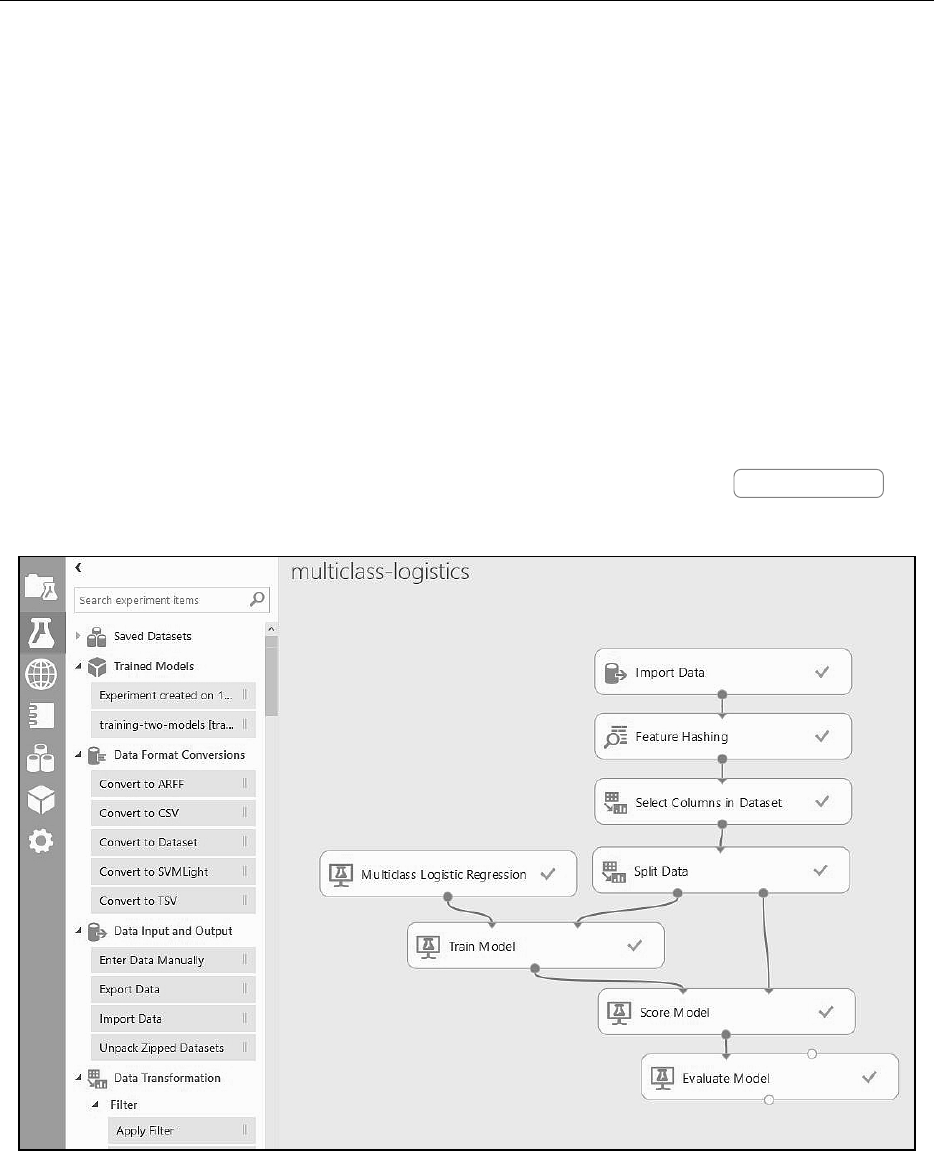10.2. Azure Machine Learning Workspace
This example also illustrates one of the challenges of the classiﬁcation problem:
science has become wonderfully multidisciplinary. The topic given for this sample
paper in arXiv is “condensed matter,” a subject in physics. Of the four authors,
however, two are in mathematics institutes and two are from physics departments,
and the abstract refers to algorithms that are typically part of computer science.
A human reader mig ht reasonably consider the abstract to be describ in g a topic in
mathematics or computer science. (In fact, multidisciplinary physics papers were
so numerous in our dataset that we removed them in the experiment below.)
Let us start with a solution in Azure ML based on a multiclass version of the
logistic regression algo rithm. Figure 10.1 shows the graph of tasks. To understand
this workﬂow, start at th e top, which is where the data source comes into the
picture. Here we take the data from Azure blob storage, where we have placed
a l arge subs et of our arXiv samples in a CSV ﬁle. Clicking the
Import Data
box
opens the window that allows us to identify the URL for the input ﬁle.
Figure 10.1: Azure ML graph used to train a multiclass logistic regression model.
The second box down,
Feature Hashing
, builds a vectorizer based on the
vocabulary in the document collection. This version comes from the Vowpal
Wabbit library. Its role is to convert each document into a numerical vector
corresponding to the key words and phrases in the document collection. This
198Chapter 10. Machine Learning in the Cloud
numeric representation is essential for the actual ML phase. To create the vector,
we tell the feature hasher to look only at the abstract text. What happens in
the o utpu t is that the vector of numeric values for the abstract text is appended
to the tuple for each document. Our tuple now has a large number of columns:
class, title, abstract, an d
vector, ..., vector[n-1]
, where
n
is the number
of features. To conﬁgure the algorithm, we select two parameters, a hashing bin
size and an n-gram length.
Before sending the example to ML training, we remove the English text of the
abstract an d the title, leaving only the class and the vector for each document. We
accomplish this with a
Select Columns in Dataset
. Next we split the data into
two subsets: a training subset and a test subset. (We specify that
Split Data
should use 75% of the data for training and the rest for testing.)
Azure ML provides a good number of the standard ML modules. Each such
module has various parameters that can be selected to tune the method. For all
the experiments described here, we just used the default parameter settings. The
Train Model
component accepts as on e input a binding to an ML method (recall
this is not a dataﬂow graph); the other in pu t is the projected training data. The
output of the Train Model task is not data per se but a trained model that may
also be saved for later use. We can now use this trained model to classify our test
data. To this end, we use the
Score Model
component, which appends another
new column to our table, Scored Label, providing the classi ﬁcation predicted by
the trained model for each row.
To see how well we did, we use the
Evaluate Model
component, which computes
a confusion matrix. Each row of the matrix tells us how the documents in that
class were classiﬁed. Table 10.1 shows the confusion matrix for thi s experiment.
Observe, for example, tha t a fair number of biology papers are classiﬁed as math.
We attrib ute this to the fact that most biology papers in the archive are related to
quantitative methods, and thus contain a fair amount of mathematics. To access
the confusion matrix, or for that matter the output of any stage in the g raph, click
on the outpu t port (the small circle) on the corresponding box to access a menu.
Selecting visualize in that menu brings up u seful information.
Table 10.1: Confusion matrix with only math, computer science, biology, and ﬁnance.
bio compsci ﬁnance math
bio 51.3 19.9 4.74 24.1
compsci 10.5 57.7 4.32 27.5
ﬁnance 6.45 17.2 50.4 25.8
math 6.45l 16.0 5.5 72
199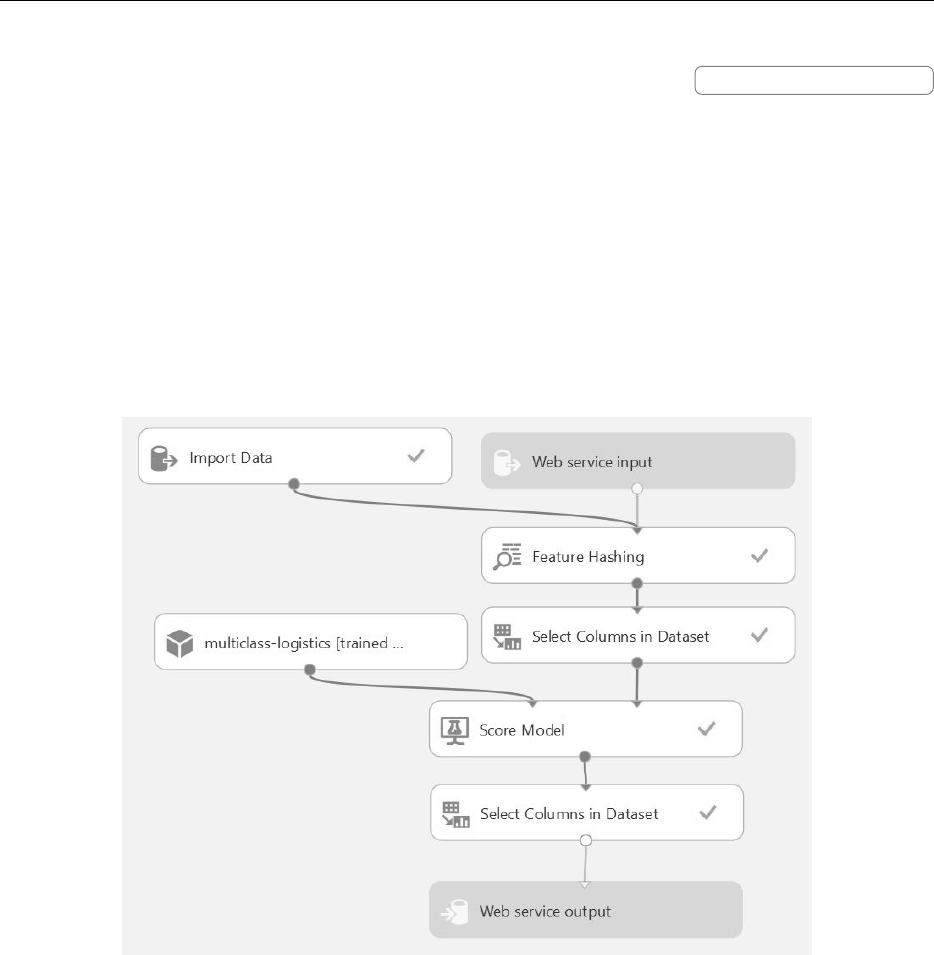10.2. Azure Machine Learning Workspace
Now that we have trained the model, we can click the
Set Up Web Service
button (not visible, but at the bottom of the page) to turn the model i nto a web
service. The Azure ML portal rearranges the graph by eliminating the split-train-
test parts and leaves just the feature hashing, column selection, and the scoring
based on the trained model. Two new nodes have been added: a web service input
and a web service output. The resu lt, with o ne exception, is shown in ﬁgure 10 .2.
The exception is that we have added a new
Select Columns
node so that we can
remove the vectorized document columns from the output of the web service. We
retain the origin al clas s, the predicted class, and the probabilities computed for
the document being in a class.
Figure 10.2: Web service graph generated by Azure ML, with an additional node to
remove the vectorized document.
You can now try additional ML classi ﬁer algorithms simply by replaci ng the
box Multiclass Logistic Regression with, for example, Multiclass Neural Network
or Random forest classiﬁer. Or, you can incorporate all three methods into a
single web service that uses a majority vote (“consensus”) method to pick the best
classiﬁcation for each document. As shown in ﬁgure 10.3, the construction of this
consensus method is straightforward: we si mp ly edit the web service graph for the
multiclass logistic regression to add the trained models for the other two methods
and then call a Python script to tie the three results together.
200